StatLect

# Posterior probability

The posterior probability is one of the quantities involved in Bayes' rule.

It is the conditional probability of a given event, computed after observing a second event whose conditional and unconditional probabilities were known in advance.

It is derived by updating the prior probability, which was assigned to the first event before observing the second event.## Definition

The following is a more formal definition.

Definition Letandbe two events whose probabilitiesandare known. If also the conditional probabilityis known, Bayes' rule gives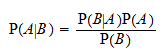The conditional probabilitythus computed is called posterior probability.

In other words, the posterior probability is the conditional probabilitycalculated after receiving the information that the eventhas happened.

## Example

Suppose that an individual is extracted at random from a population of men.

We know the following things:

• the probability of extracting a married individual is 50%;

• the probability of extracting a childless individual is 40%;

• the conditional probability that an individual is childless given that he is married is equal to 20%.

If the individual extracted at random from the population turns out to be childless, what is the conditional probability that he is married?

This conditional probability is called posterior probability and it can be computed by using Bayes' rule above.

The quantities involved in the computation are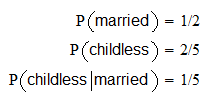The posterior probability is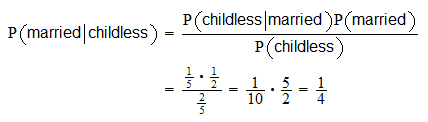## Quantities involved in the formula

There are four quantities in the formulaWe have said thatis called posterior probability.

The other three quantities are:

1. the prior probability;

2. the likelihood (or conditional probability);

3. the marginal probability.

We need to know these three quantities in order to compute the posterior.

## Law of total probability

Sometimes, we do not know the marginal probability, but we know, the likelihood of the complement of.

In those cases, we can use the law of total probability: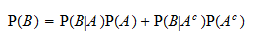where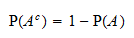## Posterior distribution

A related concept is that of a posterior probability distribution, or posterior distribution for short.

In Bayesian statistics, we assume that some observed datahave been drawn from a distribution that depends on a parameter.

In formal terms, we write this assumption as a likelihoodwheredenotes:

We assign a probability distributionto the parameter, called a prior distribution.

The prior distribution reflects our subjective beliefs or information acquired previously.

The posterior distribution is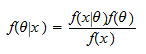The posterior distribution tells us how our prior has changed in light of the information provided by the data.

## Computation of the posterior

Thanks to its conceptual simplicity, the Bayesian approach is extremely powerful and versatile.

All we need to do is to specify a prior and a likelihood, and we face virtually no constraints in doing so.

The marginal distributionis derived from the prior and the likelihood.

We first derive the joint distribution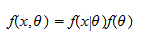and then we marginalize it to obtain the posterior.

In the continuous case, the marginal is computed by integrationIn the discrete case, it is derived by calculating a sumBoth the integral and the sum are over the whole support of.

## Closed-form posterior

There are important cases in which we are able to derive the marginalin closed form.

In those cases, the posterioris known analytically.

If we are lucky,is also a distribution whose properties (e.g., the mean and the variance) are well known.

Some examples of these fortunate cases can be found in the lectures on:

## Computational challenges

In many other cases, however, we are not able to marginalize the joint distribution because the integral (or the sum) above is intractable.

In those cases, there are numerical methods that allow us to draw Monte Carlo samples from the posterior distribution.

Such methods are discussed in the lecture on Markov Chain Monte Carlo methods.

There are also popular methods that allow us to approximate the posterior distribution with relatively simple distributions, such as mixtures of normals. These methods are called variational inference methods.

## Maximum a posteriori estimation

Moreover, we can derive interesting information about the posterior also if we do not know.

For example, we can find the Maximum A Posteriori (MAP) estimator of.

The MAP estimator, denoted by, solves the optimization problem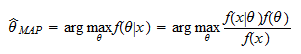which is equivalent to the problem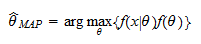We can drop the unknown denominatorfrom the objective function because it does not depend on.

The MAP estimator is the mode of the posterior distribution, that is, the value of the parameter that is most likely according to the posterior distribution.

## How to interpret the posterior

The posterior distribution is interpreted as a summary of two sources of information:

• the subjective beliefs or the information possessed before observing the data;

• the information provided by the data.

Being able to summarize these two sources of information in a single object (the posterior) is one of the main strengths of the Bayesian approach.

## How to use the posterior

What do we do after computing the posterior?

There are many things we can do. The most common are:

• plot the posterior distribution;

• calculate some summary statistics, such as the mean or the standard deviation of the posterior; this is similar to what we do in frequentist inference when we produce a point estimate of a parameter, together with a standard error of the estimate;

• find an interval or a region of space in which the true parameter has high posterior probability of being found; such intervals are known as credible intervals; this kind of exercise is the Bayesian equivalent of frequentist interval estimation.

## More details

More details about the posterior probability and posterior distributions can be found in the lectures on:

Previous entry: Parameter space

Next entry: Power function